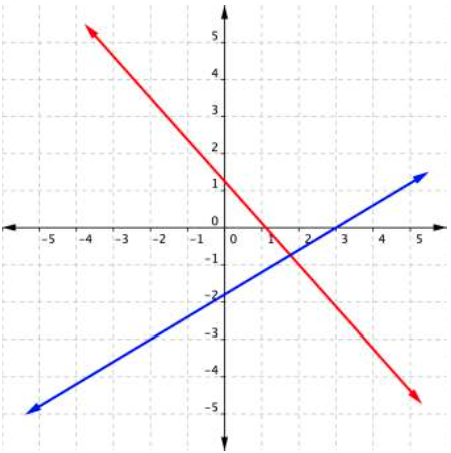## What you’ll learn to do: Use algebraic methods to solve systems of linear equations.

A graph can be used to show the solution for a system of two linear equations. However, accurately determining the solution from a graph is not always easy or accurate. For example, where do you think the two lines shown below intersect?It looks like they might intersect at $(1.8,–0.7)$—though this is only an estimate. In cases like this, you can use algebraic methods to find exact answers. One of the methods we will learn is called the substitution method. The other algebraic method for solving systems of two equations is called the elimination method.

Specifically, in this section you’ll learn how to:

• Use the substitution method
• Use the elimination method without multiplication
• Use the elimination method with multiplication
• Identify inconsistent systems of equations containing two variables
• Express the solution of a system of dependent equations containing two variables

## Contribute!

Did you have an idea for improving this content? We’d love your input.